# Ionic Solids & Lattice Energy Notes | Study Inorganic Chemistry - Chemistry

## Chemistry: Ionic Solids & Lattice Energy Notes | Study Inorganic Chemistry - Chemistry

The document Ionic Solids & Lattice Energy Notes | Study Inorganic Chemistry - Chemistry is a part of the Chemistry Course Inorganic Chemistry.
All you need of Chemistry at this link: Chemistry

MISCELLANEOUS
Ionic Bond (electrovalent bond)

It is electrostatic force of attraction between two oppositively charged ions.
Ion is formed from neutral atom by gain or loss of electrons.

Na(g)→Na+ (g) + e , Cl(g) + e- →Cl- (g)

Ionic character α Difference of group number of metal (Group IA, IIA, IIA), and non metal (group Va, VIA VIIA)

(a) Conditions for the formation of Ionic Compound:

Electronegativity difference must be large between two combining elements.
I.E. of electro-positive element should be low.
E.A. of electronegative element should be high.
Lattice energy of ionic crystal must be high.

(b) General properties of Ionic compounds.

(i) Physical state :

At room temperature ionic compuonds exist either in solid state or in solution phase but not in gaseous state.

(ii) Isomorphism

Simple ionic compounds do not show isomerism but isomorphism is their important characteristic.
Isomorphism means similar crystal structure. All alums show isomorphism because they have same general formula :

M2SO4.M'2(SO4).24H2O

(iii) Electrical conductivity:

Ionic solids are almost non-conductors. However conduct a very little amount of current due to crystal defects. All ionic solids are good conductors in molten state as well as in their aqeuous solutions because their ions are free to move.

Metallic bond:

• The electrical attraction between delocalised electrons (i.e. mobile electrons) and the positive part of the atom (i.e. kernel) is called metallic bond. Strength of metallic bond depends upon number of metallic bonds per atom.
• larger the number of metallic bonds (number of valence electrons) per atom, stronger is the metallic bonding.
• Alkali metals have only one valence electron and therefore, one metallic bond per atom and thus gives weakest metallic bonding. Hence their metling points are low.
• Alkaline earth metlas two valence electrons and form two metallic bonds per atom and therefore, gives, comparatively bit stronger metallic bonding.
• Transition metals may use innner-d-delectrons along with the outers electrons for bonding as (n - 1) d and ns have nearly same energy. So in them number of metallic bonds per atoms is quite large (more than two always). Hence their melting points are higher.
• Radius of metallic atom : Smaller the radius of atom, shorter is the bond length, hence greater is the bond strength.

Lattice Energy (Ionic Solids):

Lattice energy of a crystal is the energy released when one mole of ionic crystal is formed from gaseous ions (cations and anions).

Born Haber Cylce : Born Hable cycle is based on Hess’ law according to which the net energy change in a chemical reaction is independent of the path or the reaction and in a cyclic process, the total energy change is zero. This cycle relates the lattice energy of a crystal to other thermochemical data of energy terms involved in building a crystal lattice.
The elements in their standard state are first converted to gaseous atoms and then to ions and finally packed into the crystal lattice.
The enthalpies of sublimation and dissociation and the ionization energy are positive since energy is supplied to the system. The electron affnity and lattice energy are negative since energy is evolved in these processes.
The enthalpy of formation of (ΔHf) is the algebraic sum of the terms going round the cycle.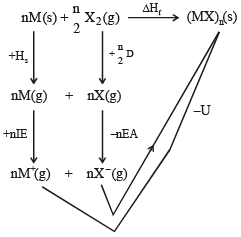Fig.  Born-Haber cycle for the formation of (MX)n (S)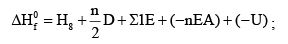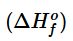= Heat of formation of (MX), (S) ;

Hs = Heat of sublimatin of metal;                  D = Dissociation energy of X2

∑IE = Ionisation energies (1E1 + 1E2 + 1E3 + ...) ; nEA = Electron affinities of nX(g) and eS

U = Lattice enrgy of crystal (MX)n(S)

All the terms except the lattice energy and electron affinity can be measured. By using known crystal structures, it was possible to calculate the lattice energy and hence values were obtained for the electron affinity.

For NaCl 381.2 = + 108.4 + 495.4  + 120.9 + EA – 757.3

hence EA = – 348.6 kJ mol–1

The cycle is used to calculate the lattice energy for unknown crystal structures.
The lattice energy, is used to calculate the solubility of the crystal. When a solid dissolves, the crystal lattice must be broken up. The ions so formed are solvated (with the evolution of energy). When the latticer energy is high a large amount of energy is required to break the lattice.

Factors affecting formation of ionic crystal : It is favoured by

1. Low heat of sublimation of metal.
2. Low I.E. of metal.
3. Low heat of dissociation of X2
4. High E.A. of X
5. High lattice energy of crystal formation

Application of Born Haber Cycle:

Formation of NaCl(s) : Energy (kJ mol–1)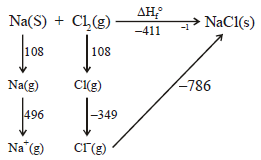Lattice energy, U, is the characteristic property of ionic crystals. It can be thoretically calculated by Born equation and depends upon ionic charge, ionic radii, crystal structure and electronic configuration of ions.

In an ionic crystal at least two forces are operating.
(i) the electrostatic attractive force (or attractive potential energy) acting between the oppositely charged ions and

(ii) Interatomic repulsive force (or repulsive potential energy). The variation of potential energy (PE) of oppositely charged ions viz M+ and X with their inter-nuclear separation (r) in the ionic crystal,
M+X.

The lattice energy of a crystal may be defined at the decrease in energy that takes place in the process of bringing the ions from an infinite distance to their equilibrium position in the stable lattice. In other words the lattice energy of an ionic crystal M+ X may be defined as the energy released when the correct number of gaseous cations M+ and anions X are brought together from an infinite distance to form one gram mole of the solid crystal.

M+(g) + X(g) → M+X- (s) + Energy released (Lattice energy, U)

Lattice energy is defined as the energy required to remove ions of one gm mole of a solid ionic crystal from their equilibrium position in the crystal to infinity.

M+X (x) (inic crystal) + Energy required → M+(g) + X- (g) · The lattice energy of an ionic crystal is determined by colombic interaction between all its ions attractive forces acting between the ions of opposite charges and repulsive forces which result from the interpenetration of their outermost electron clouds.

Born-Lande equation:

When the ions approach each other, the only force acting at first between them is the attractive force which directly proportional to the product of the charges carried by the cation and anion and inversely proportional to the distance of their separation. Thus the attractive force [or potential energy, (PE)attraction] is given by :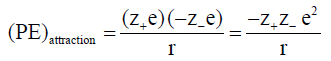... (i)

Here z+ e and z e = charges on the cation and anion, e = charge on the electron, z+ and z =
positive integers
and r = distance between the cation and the anion.
As the ions come very close to each other, they repel one another because of interpenetration of
their electron clouds.
The repulsive force [or repulsion energy, (PE)repulsion] is given by :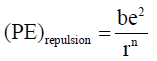.....(ii)

where n and b are called Born exponent and repulsion coefficient respectively.
When the ions are brought together from an infinite distance the total potential energy (PE)total is
given by

(PE)total = (PE)attraction + (PE)repulsion
So, the lattice energy, U, defined as the energy released in the formation of a mole of an ionic crystal from the gaseous ions which are at infinite distance from each other. Thus if N is the Avogadro’s number, then by definition.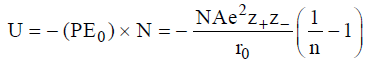....(iii)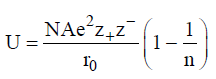....(iv)

here A = madelung constant n = born exponent
The lattice energy varies directly as the product of the charges on the ions of the ionic crystal. Thus
the lattice energies of ionic crystals containing uni-univalent (e.g. Li+F), uni-bivalent (e.g. Ca2+ F+)
and bivalent (eg. Mg+2S–2) ions are in the decreasing order LiF (U = – 240 kcal/mol) < Ca+2 F(U
= – 623) < Mg2+S2– (U= – 777)

(iii) The lattice energy varies inversely as the inter-nuclear distance, r0 between the oppositely charged
ions in an ionic crystal.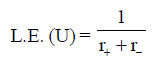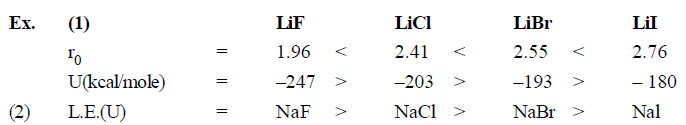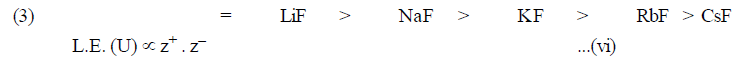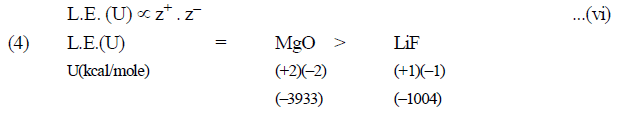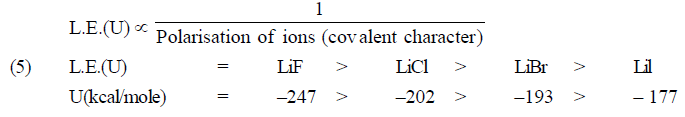Covalent character in ionic compound (Fajan’s rule)

• There No compound has 100% ionic character.
• Covalent character in ionic compound can be explained with the help of Fajan’s rule.
• According to Fajan’s rule covalent character increases if
 Cation Anion (a) Small size (i) Large size (b) More charge (ii) More charge (c) Pseudo inert gas configuration of cation

Factors (Polarisation)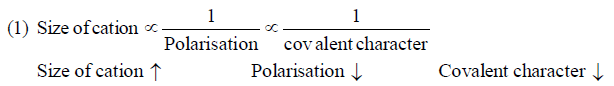Covalent Character BeCl2 > MgCl2 > CaCl2 > SrCl2 > BaCl2

Size of Anion ∝ Polarisation ∝ Covalent character

Size of Anion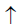PolarisationCovalent characterLiF < LiCl < LiBr < Lil

(3) Charge on Cation & Anion ∝ Polarisation ∝ Covalent character
NaCl < MgCl2 < AlCl3
AlF3 < Al2O3 < AIN

(4) Cation which has pseudo inert gas configuration, shows more polarising power in comparision of
cation that has inert gas configuration CuCl > NaCl (Covalent character)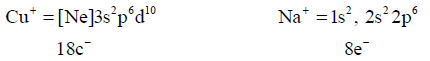Pseudo inert gas configuration                            Inert gas configuration

Note: Covalent character of cation
NIG configuration > PIG configuration > IG configuration
Example: PbCl2 > CdCl2 > CaCl2

The document Ionic Solids & Lattice Energy Notes | Study Inorganic Chemistry - Chemistry is a part of the Chemistry Course Inorganic Chemistry.
All you need of Chemistry at this link: ChemistryUse Code STAYHOME200 and get INR 200 additional OFF

## Inorganic Chemistry

49 videos|71 docs|16 tests

Track your progress, build streaks, highlight & save important lessons and more!

,

,

,

,

,

,

,

,

,

,

,

,

,

,

,

,

,

,

,

,

,

;